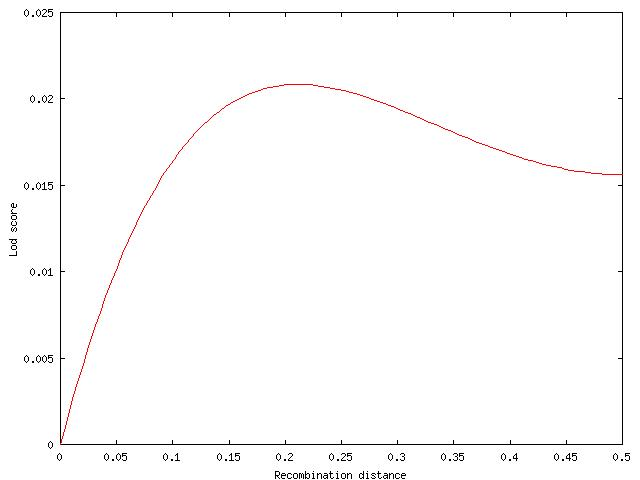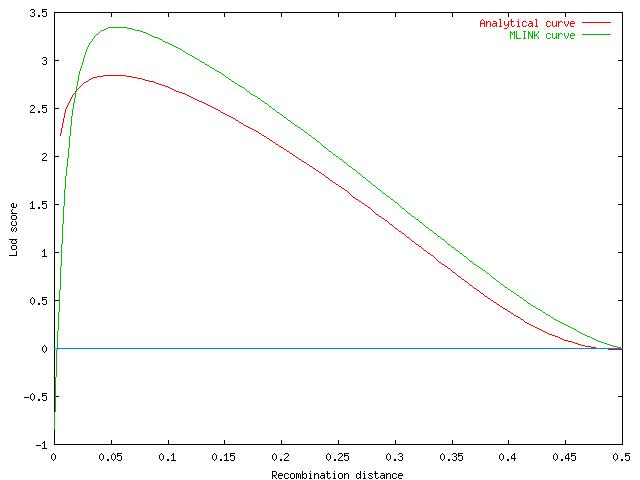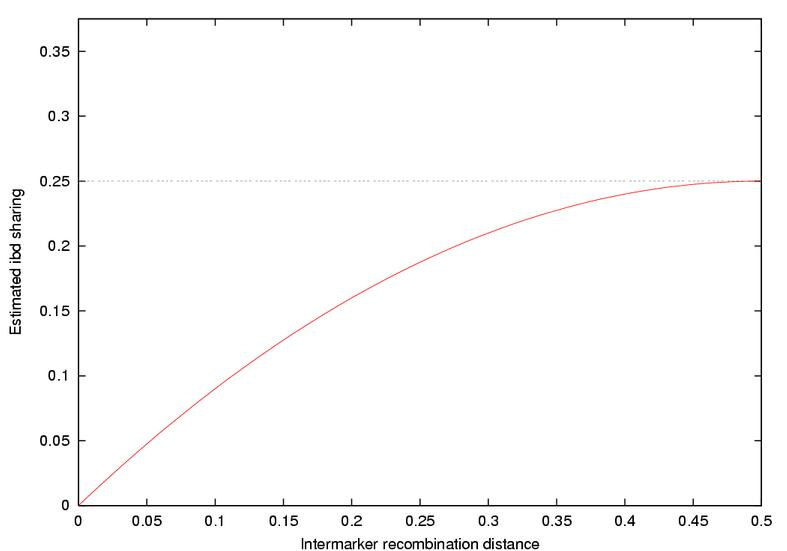## Likelihood

We will partly follow the organisation of Ott's book (1985, 1991). The likelihood is the probability of observing particular data given a specific value of the parameter(s) of the underlying hypothesized statistical process (probability distribution function). The usual likelihood used in linkage analysis is the binomial as applied to the recombination fraction. In the phase-known fully informative case, we can determine if a recombinant or nonrecombinant event occurred between two loci in the formation of a parental gamete. The likelihood is,

Pr(observe a recombinant) = c ,

where c is the recombination fraction. One realises that in the case that c is unknown, this probability is not the same as the "true" probability (in the frequentist sense) we would quote if c was known -- why the term likelihood is preferred. If we had a sibship of four children, three recombinants and one nonrecombinant then, we would write,

L(R,R,R,NR; c) = c3(1-c) ,

where L() makes the difference between probability and likelihood explicit. Furthermore, there are an infinite number of possible values for the likelihood for given data. We usually will plot a likelihood curve in the case that there is one parameter eg c, or a surface in the case there are two or more parameters eg cm and cf. For mathematical convenience, many formulae are expressed in terms of the log of the likelihood.

## The lod score

Previously, we introduced the likelihood ratio as a means for comparing two suggested values of a parameter based on particular observed data. In the case of linkage analysis, one natural hypothetical value is that under the sensible null hypothesis that the two loci are unlinked, c=50%. The lod score is the decimal log likelihood ratio,

Z(x) = log10[ L(c=x) / L(c=0.5) ]

The likelihood of our observed three recombinants and one nonrecombinant given that the recombination fraction was 50% is,

L(R,R,R,NR; c=0.5) = L(0.5) = 0.54 ,

so,

Z(c)=log10[16(1-c)c3] .

The classical critical lod score of 3 therefore can be interpreted as stating the estimated value of c (say we choose c=10%) is 1000 times more likely than the null hypothesis that c=50% for the given (marker and pedigree) data. If a lod score of -2 was observed, then the null hypothesis that c=50% is 100 times more likely than the alternative c=10%.

Lod scores are a popular summary of a likelihood because they are easily added (representing multiplication of the "raw" likelihoods) across observations and pedigrees. In the fully informative case, a recombinant contributes a lod score of Z(2c), and a nonrecombinant, Z(2-2c). A disconcerting property is that the lod score can be infinite (where a contributing likelihood is zero), for example Z(c=0) with the observed number of recombinants greater than zero.

## Maximum likelihood estimation

In the case that c is unknown, but we have observed a certain number of recombinants and nonrecombinants, the maximum likelihood estimate (MLE) of c is that value that gives rise to the largest value of the likelihood (or lod score). In the case that the likelihood surface is simple in shape, it is possible to calculate this analytically, but mostly numerical methods are needed. Fans of the MLE know that it has several advantages over other estimators.

The MLE is usually the most efficient, in the sense of the precision obtained using a given set of data, and the most consistent, in that with increasing sample size it will converge closer and closer to the true value. It is also asymptotically (large sample) unbiased. For small samples, it can be more biased than other less efficient estimators. That is, the MLE value might tend to be lower or higher than the true value.

A nice example is given by Ott. The MLE for the recombination fraction in the phase-known, fully informative case is,

c* = R/(R+NR)

where R is the number of observed recombinants, and NR the number of observed nonrecombinants. This is the "direct estimate" of c we are familiar with already, and is unbiased. The MLE of the square of the recombination fraction, logically is,

MLE(c2) = [c*]2

It turns out this estimator is biased upwards, in that the expected value for this is actually,

E{[c*]2} = c2 + c(1-c)/[R+NR]

## Phase-unknown testcross with two offspring: codominant marker loci

We have previously seen how to estimate c in the case of two offspring from the testcross.

 First Child SecondChild AB or ab Ab or aB AB or ab (c2+(1-c)2/2 c(1-c) Ab or aB c(1-c) (c2+(1-c)2/2

The sib-pair is concordant or discordant for type. The lod score contributed by a pair is,

 concordant: log10[2-4c(1-c)] , discordant: log10[4c(1-c)].

## An example of lod score linkage analysis for a nuclear family with three offspring

Ott describes an example where the lod score contributed by a larger family is calculated:

Genotypes at TC2 and HLA-A

```TC2          1 3       1 3
HLA-A        a b       c d
1         2
|         |
+----+----+
|

+--------+--------+
|        |        |
1 1      1 1      1 3
a c      a c      a c
3        4        5
```

The likelihood for this family is:

L(c) = c(1-c)[1-3c(1-c)]/4.

This formula arises from the fact that both parents are phase unknown, as is the individual 5. Each of the eight possible arrangements is equally likely:

 Person 1 Person 2 Person 5 Recombinants Likelihood 1a/3b 1c/3d 1a/3c NR,NR,NR,NR,NR,R c(1-c)5 1a/3b 1c/3d 1c/3a NR,NR,R,NR,NR,NR c(1-c)5 1a/3b 1d/3c 1a/3c NR,NR,NR,R,R,NR c2(1-c)4 1a/3b 1d/3c 1c/3a NR,NR,R,R,R,R c4(1-c)2 1b/3a 1c/3d 1a/3c R,R,R,NR,NR,R c4(1-c)2 1b/3a 1c/3d 1c/3a R,R,NR,NR,NR,NR c2(1-c)4 1b/3a 1d/3c 1a/3c R,R,R,R,R,NR c5(1-c) 1b/3a 1d/3c 1c/3a R,R,NR,R,R,R c5(1-c)

The lod for the family is the average of these eight possibilities. It reaches its maximum value Zmax at c=0.21.## A more complicated example from Morton (1956)

This is from a classic paper demonstrating the methods, and also one of the first examples of testing for homogeneity of linkage in defferent pedigrees.

Pedigree 5 from Lawler and Sandler (1954) used by Morton (1956).

```
101     102
|       |
+---+---+
|
+--------------------+----------------------------------------+
|                                                             |
201   202                                                     203   204
|     |                                                       |     |
+--+--+                                                       +--+--+
|                                                             |
+--------+--+------------+--------+------------------+           +-----+-----+
|           |            |        |                  |           |     |     |
Aff   UnA   Aff     ?    UnA      Aff  UnA      ?    Aff   UnA   Aff   UnA   Aff
1 3   x x   1 3    x x  3 3       1 3   x x    x x   1 3   x x   1 2   2 3   3 3
301   302   303    304  305       306   307    308   309   311   310   312   314
|     |     |      |              |     |      |     |     |     |
+--+--+     +---+--+              +--+--+      +--+--+     +--+--+
|            |                    |            |           |
|    +----+--+-+----+         +---+---+    +---+-----+     |
|    |    |    |    |         |   |   |    |         |     |
UnA  UnA  Aff  Aff  Aff   ?   Aff Aff UnA  Aff   ?   Aff   UnA
3 3  2 3  1 1  1 2  1 1  x x  1 1 1 3 3 3  1 3  x x  1 3   2 2
405  407  408  409  410  412  411 413 414  415  417  418   419
|    |                 |    |
+--+-+                 +--+-+
|                      |
|                  +---+---+
|                  |   |   |
Aff                Aff Aff Aff
1 3                1 1 1 1 1 1
504                505 506 507
```

One needs to know that familial elliptocytosis is extremely rare, so that the population allele frequency of the disease allele is very low. Examination of this pedigree and others shows the inheritance is consistent with fully penetrant autosomal dominant inheritance. These two assumptions allow us to score each affected person as Dd, each unaffected person as dd, and take each D allele as being identical by descent. Also, the allele frequencies for the marker locus (Rhesus blood group) are well known, so for individuals who are untyped, we can weight the possibilities appropriately (0.4076, 0.1411, 0.3886,0.0627).

Morton (1956) gives the lod score expression for this family as:

Z = log10 220/39168 {810c(1-c)19 + 324c(1-c)18 + 180c(1-c)17 + 72c(1-c)16 + 90c3(1-c)17 + 72c3(1-c)16 + 40c3(1-c)15 + 24c3(1-c)14 + 90c4(1-c)15 + 20c4(1-c)13 + 90c5(1-c)15 + 432c5(1-c)14 + 20c5(1-c)13 + 104c5(1-c)12 + 1800c6(1-c)14 + 558c6(1-c)13 + 440c6(1-c)12 + 176c6(1-c)11 + 90c7(1-c)13 + 324c7(1-c)12 + 120c7(1-c)10 + 360c8(1-c)12 + 378c8(1-c)11 + 80c8(1-c)10 + 76c8(1-c)9 + 4c8(1-c)4 + 180c9(1-c)11 + 522c9(1-c)10 + 80c9(1-c)9 + 100c9(1-c)8 + 10c9(1-c)3 + 180c10(1-c)10 + 846c10(1-c)9 + 40c10(1-c)8 + 216c10(1-c)7 + 18c10(1-c)4 + 4c10(1-c)2 + 1170c11(1-c)9 + 378c11(1-c)8 + 260c11(1-c)7 + 72c11(1-c)6 + 45c11(1-c)3 + 180c12(1-c)8 + 396c12(1-c)7 + 40c12(1-c)5 + 18c12(1-c)2 + 270c13(1-c)7 + 234c13(1-c)6 + 40c13(1-c)5 + 52c13(1-c)4 + 180c14(1-c)6 + 108c14(1-c)5 + 80c14(1-c)4 + 16c14(1-c)3 + 90c15(1-c)5 + 162c15(1-c)4 + 20c15(1-c)3 + 180c16(1-c)4 + 72c16(1-c)3 + 90c17(1-c)3}

The reported peak lod score in the paper was 3.31 at a recombination distance of approximately 5% (this may be an error as the equation above has a maximum value of only 2.84; MLINK gives a lod score of 3.40 at c=0.05).## The Elston-Stewart algorithm for general pedigrees

The lod score formulae for larger pedigrees are difficult to generate and evaluate. This is especially the case where some pedigree members are untyped, or the relationship between phenotype and genotype is not the direct relationship of codominant loci. Certain computer programs (actually computer algebra systems) can write out these high order polynomials, and then evaluate them. The standard programs such as the LINKAGE programs, CRI-MAP, MENDEL and GENEHUNTER, do not produce a single closed form expression. They instead numerically evaluate the likelihood in a recursive fashion.

For even large pedigrees that meet certain criteria (absence of loops, no more than one founder x founder mating), it is possible to write the likelihood in a form,

L(c) = Sum Pr(xi|gi)Pr(gi|parents,c)... Sum Pr(xn|gn)Pr(gn|parents,c)

where,

xi is the phenotype of the ith individual,

gi is the (poly-)genotype of the ith individual,

Pr(gi|parents) is the probability of observing that genotype given the parental genotypes (the population genotype frequencies in the case of founders), and the recombination distance between the loci contributing to the genotype,

the summation for each individual is over all possible genotypes consistent with their phenotype (eg two possibilities for the phase-unknown case, two codominant loci), and the individuals are ordered by their position in the pedigree, from founders downwards (to descendants). The nested sums are evaluated from right to left, so the likelihood of the descendants below a particular individual become summarised in the likelihood of the individual.

For pedigrees where loops or multiple founder matings exist, the more complicated pedigree traversal algorithms used in the LINKAGE programs must be used. Given the complexity of evaluating the lod score for large pedigrees, values are usually produced for a grid of fixed values, such as c=(0.0,0.01,0.05,0.1,0.2...). Ott gives formulae that are useful for interpolating the full lod curve from such values. Asymptotically, lod curves approach a Gaussian shape, and so fitting a Gaussian curve to the known lod scores is an efficient method. This is performed by the programs VARCO3 and VARCO6 distributed among the LINKAGE utilities.

## Confidence intervals for the recombination fraction

The lod score curve can also be used to give a confidence region for c. The easiest method is the "1 unit" confidence interval or "support interval". This is constructed by taking the closest values of c on either side of Zmax which have a lod score of Zmax-1. The steepness of the lod curve around the MLE does reflect the precision of the estimate, and asymptotically, this steepness measured as the second derivative of the likelihood function gives the sampling variance of the estimate (as the inverse of the Fisher information). An alternative approach is to use the VARCOx programs and their Gaussian approximations to give asymptotically correct confidence intervals.

## Multipoint estimation of identity-by-descent sharing

Programs such as Genehunter use maximum likelihood approaches to improve the estimation of ibd probabilities when genotypes at multiple linked markers are available. If the two markers in the family below were unlinked (c=0.50), then the mean ibd sharing for the siblings would be 0.25 at A and 0.0 at B (using the results from the last chapter).

Genotypes at two neighbouring marker loci

```Marker A     1 1       1 2
Marker B     a b       c d
1         2
|         |
+----+----+
|
+----+----+
|         |
1 1       1 2
a c       b d
3         4
```

If the markers were linked, then we can use the joint likelihood to improve the ibd estimation at the first, less informative, marker. Denoting the "1" alleles for person 1 as "p" and "m" to denote phase:

Person 1Person 2Person 3Person 4RecombinantsLikelihoodibd(A)ibd(B)
pa/mb1c/2dpa/1cpb/2dNR,NR,R,NR c(1-c)3 0.50.0
pa/mb1c/2dpa/1cmb/2dNR,NR,NR,NR(1-c)4 0.00.0
pa/mb1c/2dma/1cpb/2dR,NR,R,NR c2(1-c)20.00.0
pa/mb1c/2dma/1cmb/2dR,NR,NR,NR c(1-c)3 0.50.0
pa/mb1d/2cpa/1cpb/2dNR,R,R,R c3(1-c) 0.50.0
pa/mb1d/2cpa/1cmb/2dNR,R,NR,R c2(1-c)20.00.0
pa/mb1d/2cma/1cpb/2dR,R,R,R c4 0.00.0
pa/mb1d/2cma/1cmb/2dR,R,NR,R c3(1-c) 0.50.0
ma/pb1c/2dpa/1cpb/2dR,NR,NR,NR c(1-c)3 0.50.0
ma/pb1c/2dpa/1cmb/2dR,NR, R,NR c2(1-c)20.00.0
ma/pb1c/2dma/1cpb/2dNR,NR,NR,NR(1-c)4 0.00.0
ma/pb1c/2dma/1cmb/2dNR,NR,R,NR c(1-c)3 0.50.0
ma/pb1d/2cpa/1cpb/2dR,R,NR,R c3(1-c) 0.50.0
ma/pb1d/2cpa/1cmb/2dR,R,R,R c4 0.00.0
ma/pb1d/2cma/1cpb/2dNR,R,NR,R c2(1-c)20.00.0
ma/pb1d/2cma/1cmb/2dNR,R,R,R c3(1-c) 0.50.0

Therefore, the ibd sharing for marker B is 0.0, but that at marker A is now [c(1-c)3+c3(1-c)]/[1-2c(1-c)], where c is the distance between the markers. This estimate ranges from 0.0 to 0.25, as shown in the graph below.For practical applications involving many markers, the likelihood can be factored out in terms of a Markov model incorporating the ibd at a marker, along with the transition probabilities from marker to marker.